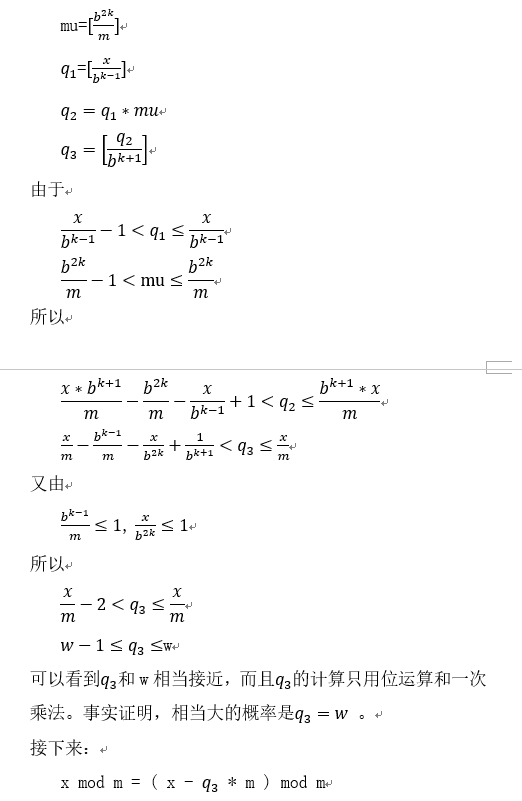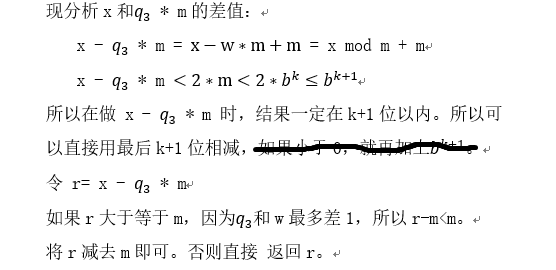# 大数取模运算Barrett reduction

Barrett reduction算法的证明和使用。

m的位数是k

q1 = x / b^(k-1)

q2 = q1 * mu

q3 = q2 / b^(k+1)

r1 = x % b^(k+1)

r2 = (q3 * m) % b^(k+1)

r = r1 - r2

if ( r > m ) r -= m

return r

[]表示取整，b是大数的进制